Tuesday, March 13, 2012

ပ်ားအိမ္၏ ပံုသ႑ာန္ႏွင့္ ဆ႒ဂံ၏ ေထာင့္က်ဥ္းဆိုေသာ အယူအဆ [ပ်ားအိမ္မ်ား အဘယ္ေၾကာင့္ ဆ႒ဂံပံု ႐ွိၾကသနည္း ၏ ေနာက္ဆက္တြဲ

၁၈ ရာစုခန္႔တြင္ ျပင္သစ္ပညာ႐ွင္တစ္ဦးသည္ ပ်ားအိမ္ကို ေပတံျဖင့္ တိုင္းထြာခဲ့ဘူးသည္။ ထိုကဲ့သို႔ တိုင္းထြာရာတြင္ စိတ္ဝင္စားဖြယ္ေကာင္းေသာ အေၾကာင္းအရာတစ္ခုကို ေတြ႕႐ွိခဲ့သည္။ ဆ႒ဂံပံု႐ွိ ပ်ားအိမ္ေပါက္မ်ား၏ ေထာင့္ေျခာက္ေထာင့္သည္ တူညီေသာ စည္းမ်ဥ္းကို လိုက္နာလ်က္ ေထာင့္က်ယ္သည္ ၁၀၉° ၂၈' ၊ ေထာင့္က်ဥ္းသည္ ၇၀° ၃၂' ႐ွိသည္ကို ေတြ႕႐ွိရသည္။

ဖာ္ျပပါစာကို ၂၀၁၂ခု ေဖ (၂၇) ေန႕က ပို႔စ္တင္ၿပီးကတည္းက စိတ္ခုေနခဲ့တယ္ပ်ားအိမ္ပံုေတြ လိုက္႐ွာၾကည့္ေသာ္လည္း ေထာင့္က်ဥ္းပါတဲ့ ဆ႒ဂံပံု မေတြ႕ခဲ့။ မယံုရင္ ပံုျပင္လို႔ မွတ္ရမလို ျဖစ္။ အခုေတာ့ ေတြ႕ပါၿပီ။ ပ်ားအိမ္ ဆ႒ဂံပံုရဲ႕ ေထာင့္က်ဥ္းကို ဒီကရီ ဘယ္ေလာက္႐ွိသလဲ တြက္ခိုင္းတဲ့ ပုစာၦတစ္ပုဒ္ ... archimedes-lab.org မွာ...။

ခက္ခဲမႈအဆင့္က အျမင့္ဆံုးဆိုပဲ။ အေျဖမွန္ ေျဖႏိုင္သူ တစ္ဦးမွ် မ႐ွိဆိုပဲ။ ဂ်ီၾသေမႀတီ ညံ့တဲ့ က်ေနာ့္အတြက္ ပံု နဲ႕ ႐ွင္းျပခ်က္ကို ၾကည့္ရင္းကကို လည္ထြက္ေန...။ ဘာပဲျဖစ္ျဖစ္ ကိုယ္တင္တဲ့ ပို႔စ္ပါ ကြန္းတန္႕က ကိုယ့္တာဝန္ဆိုေတာ့ ပူးတြဲထားလိုက္တာ ေကာင္းမယ္ ယူဆလို႕
တလက္စတည္း ေျဖ႐ွင္းခ်က္ကေလးပါ ဆက္တင္လိုက္ပါရေစခင္ဗ်ား...။ ထံုးစံအတိုင္း Credited to စာပိုင္႐ွင္ http://www.archimedes-lab.org/monthly_puzzles_72.html ေပါ့ေနာ္း

Puzzle # 117 Difficulty level: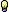, general math knowledge.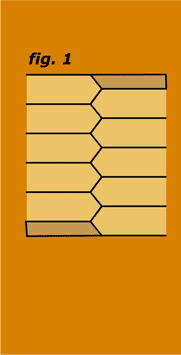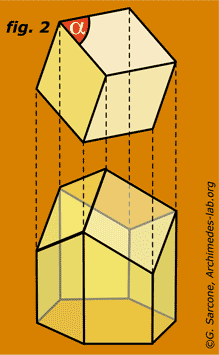The Geometry of the Bees...

The shape of the wax cells is such that two opposing honeycomb layers nest into each other, with each facet of the closed ends being shared by opposing cells, and with the open ends facing outward, as illustrated in fig. 1 above. Each cell is actually a rhombic decahedron, that is an hexagonal prism having three rhombi at its closed end (as shown in fig. 2 above). In short, each cell is a ten-sided structure with one side open. Mathematicians made extensive studies of the isoperimetric properties of the honeycomb cells and believed them to be the most efficient design possible. If the faces of every cell contain the LARGEST possible volume with the LEAST possible surface, what is the value of the angle alpha? The faces of the hexagonal prism are each 1-unit wide...

Key words: isoperimetric, rhombic decahedron, rhombus, hexagonal prism.

Related puzzles:Square vs rectangle problem.The best cardboard box.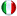Italiano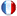Français

Source of the puzzle:
©G. Sarcone, "Il Calcolo Differenziale ed Integrale", by Ing. Gustavo Bessière, Hoepli Publications, page 114.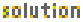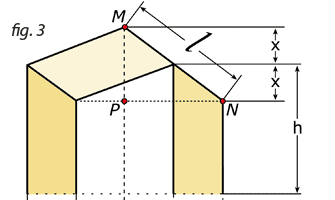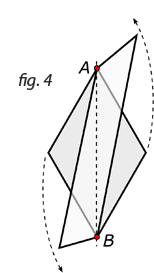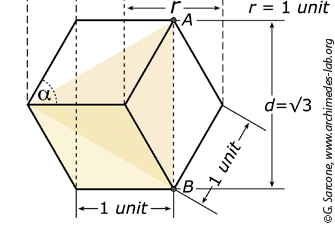a) l is the hypotenuse of the right triangle MNP shown in fig. 3 above, then:
l2 = (2x)2 + 12 and
l = √(4x2 + 1)

b) The diagonal AB of the rhombus at the closed end of the cell does not depend upon its spatial angulation (the axis AB remains the same even when the spatial angle changes, fig. 4) but upon the sides of the hexagonal prism and the radius r that circumscribes the hexagonal section. Thus the length of AB is:
2√[12 - (1/2)2] = √(4 - 4/4) = √3

c) So, the area of each of the 3 rhombi at the closed end of the cell is:
dl/2 or
√3 √(4x2 + 1)/2

d) The area of each lateral trapezoid of the prism is:
1/2[h + (h - x)] · 1 unit, or
(2h - x)/2 units

e) Therefore, the whole surface of the cell is:
S = 3[√3 √(4x2 + 1)/2] + 6(2h - x)/2 units
Reducing to
S = 3/2[√3 √(4x2 + 1)] + 6h - 3x units

f) To know where the variable x peaks we take the derivative of S at the point 0:
S' = 3/2[8√3 / 2√(4x2 + 1)] - 3 = 0

so
6x(√3) / √(4x2 + 1) = 3
and finally
x2 = 1/8

g) If we replace x2 in the first equation a) with this last value, we obtain:
l = √(4x2 + 1) = √[4(1/8) + 1] = √1.5

The ratio of both diagonals of the rhombus gives us the tangent of the semi-angle α of the rhombus:
l/d = tg α/2 = √1.5/√3

Thus, the angle α of the rhombus at the closed end of the cell is:
2 arctg √1.5/√3 = 70.52877937.... ≈ 70° 31"
(arc = Inverse trigonometric functions)The 5 Winners of the Puzzle of the Month are:
No winners... Yes, the puzzle was a little bit harder.

Sorry!

More Math Facts behind the puzzle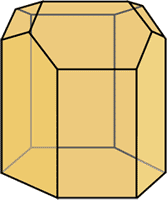Curiously, a 3-dimensional honeycomb partition is not optimal! In 1965, the Hungarian mathematician László Fejes Tóth discovered that a cell end composed of two hexagons and two smaller rhombi (see fig. opposite), instead of three rhombi, would actually be 0.035% (or approximately 1 part per 2850) more efficient. But, frankly, this difference is too minute to measure on an actual honeycomb, and irrelevant to the hive economy in terms of efficient use of wax, and because the honeycomb walls have a definite thickness, it is not clear that Tóth's structure would indeed be an improvement... In that respect, the honeycomb is more like a wet foam than a dry foam. Several years ago, the physicist D. Weaire and his colleague R. Phelan undertook to construct two-layer foams with equal-sized bubbles, and they found that the dry foams did take on Tóth's pattern. But when they gradually added liquid to thicken the bubble walls, something 'quite dramatic' happened: the structure suddenly switched over to the three-rhombus configuration of a honeycomb (it seems, then, that the bees got it right after all!). The switch also occurs in the reverse direction as liquid is removed.

Credit and many Thanks to : http://www.archimedes-lab.org/monthly_puzzles_72.html
Lu Zein